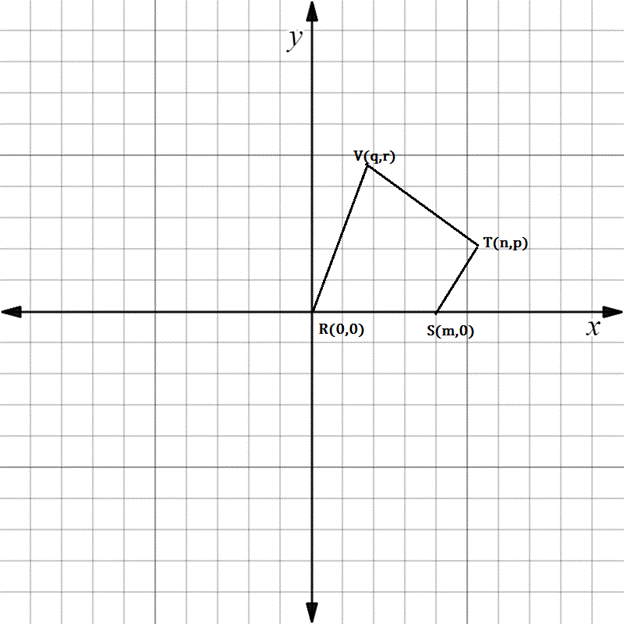Chapter 10.3, Problem 26EElementary Geometry For College St...

7th Edition
Alexander + 2 others
ISBN: 9781337614085

Solutions

Chapter
SectionElementary Geometry For College St...

7th Edition
Alexander + 2 others
ISBN: 9781337614085
Textbook Problem

In Exercises 23 to 28, find the equation (realtionship) requested. Then eliminate fractions and square root radicals from the equation.For quadrilateral R S T V , suppose that R V - ║ S T - . State an equation that relates m ,   n ,   p ,   q and r .To determine

To state:

An equation that relates m,n, p, q and r for the given figure.

Explanation

The given figure is shown below.

It is given that RVST.

If RV is parallel to ST, then their slopes are equal.

That is mRV=mST

Finding the slope of RV.

x1,y1=R0, 0

x2,y2=Vq, r

Formula for slope of the line containing two points.

Slope is denoted by m.

m=y2-y1x2-x1

Here x1x2

Substituting the x and y co-ordinates.

mRV=r-0q-0

Simplifying,

mRV=rq

Finding the slope of ST

Still sussing out bartleby?

Check out a sample textbook solution.

See a sample solution

The Solution to Your Study Problems

Bartleby provides explanations to thousands of textbook problems written by our experts, many with advanced degrees!

Get Started